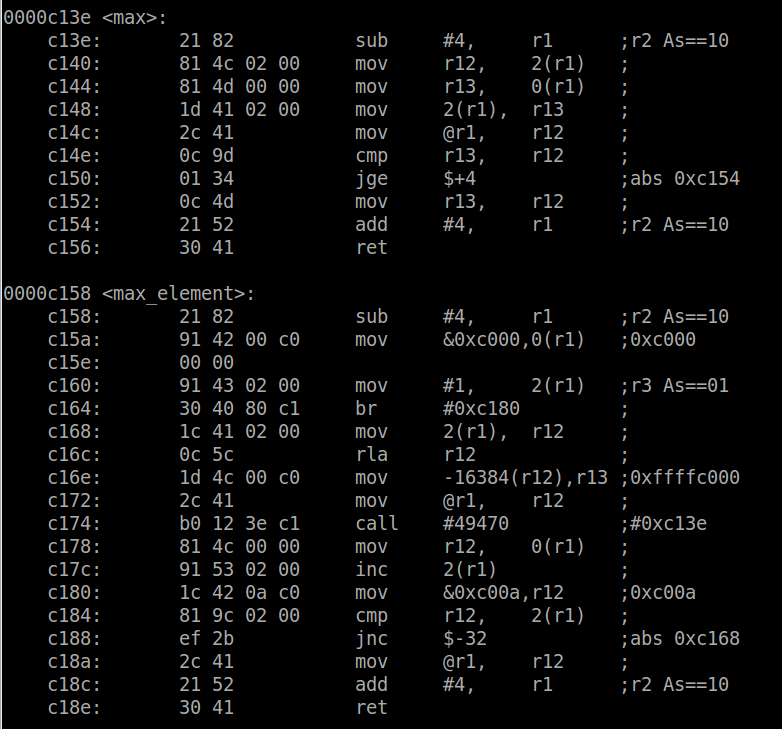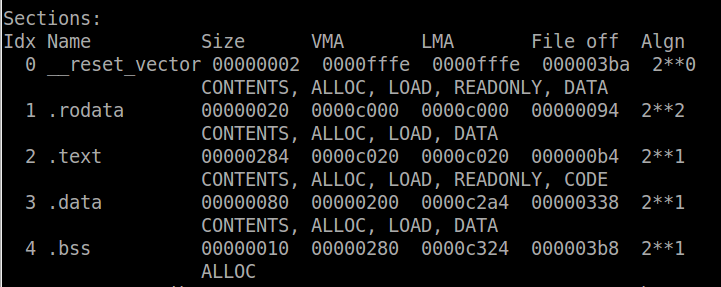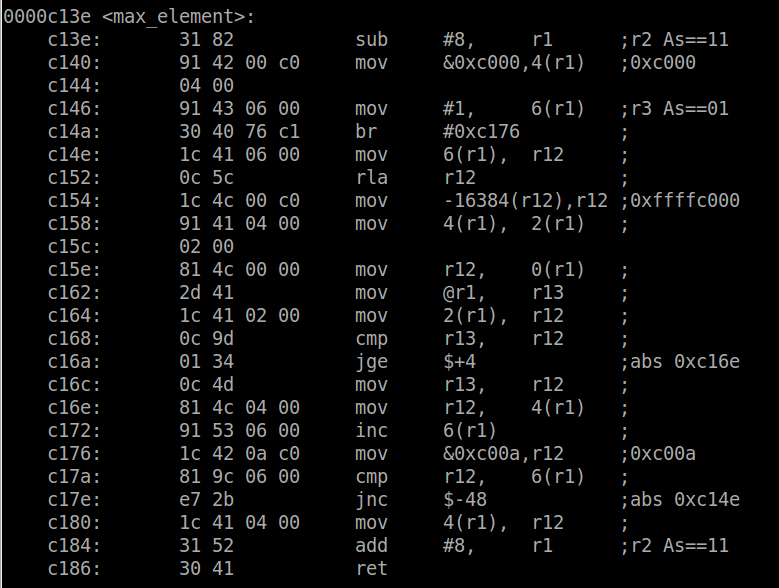# Battle of the Standards: C90 vs. C99 – Round 3Inline Functions

Before the C programming language was created and engineers programmed in assembly, optimizing code was a big part of the daily routine. Today, optimization is less the job of the engineer as it is the responsibility of the compiler. Most will agree that when writing code, readability and maintainability takes precedence over fancy or confusing optimizations. Engineers should only take optimization into their own hands when absolutely necessary. Modern compilers are much more intelligent than they used to be and are able to make ‘decisions’ on how to optimize code for speed and size. To help compilers with some of these decisions, the C99 standard introduced the inline keyword which suggests to the the compiler that the body of that function should be placed inline wherever it is called. What does this mean? Let’s take a deeper look.

A common ‘solution’ to these issues is to use macros instead of functions. Code defined by a macro is always executed inline. The precompiler searches the translation unit (C file) for instances of the macro name and replaces them with the defined code. This makes macros much faster and efficient in general than functions because there is no overhead, require no additional stack space and and can be better optimized by the compiler. Macros are not typed making them more flexible, but this can also be a double edge sword. Multi-line macros are more difficult to read than functions, and there are are other caveats like side-effects, cryptic compiler errors, and code bloat. Code bloat is when macros are scattered everywhere throughout the code. Since the same code is repeated over and over again each time the macro is called, additional program memory must be allocated. For example, if a macro compiles down to 10 bytes of machine code and is used 1000 times, that’s 10000 bytes of program memory required. If a function was used instead, there might be some function overhead, but the body of the function would only occupy 10 bytes of program space. Finally, macros (specifically function-like macros) are often frowned upon my many coding standards. The general rule of thumb is use macros to replace functions only when performance is an issue. However this is a broad generalization, and when a code base becomes big and complex, it may not necessarily be the best solution.

When C99 introduced inline functions, it tried to address the caveats of both functions and macros providing the best of both worlds while catering to the increasingly complex code being produced. Inline functions provide all the benefits of a normal function, but leave it up to the compiler to determine what is the best way to optimize the code – i.e. to inline or not to inline. This is based on a number of code characteristics as defined by the compiler algorithm and the compiler optimization level used. Inline is a keyword just like static or volatile. The difference with the inline keyword is that it is just a suggestion to the compiler. It does not have to inline the function just because a function was declared as such. By pointing out which functions the compiler might want to optimize, the compiler knows which functions to focus its optimization efforts on while keeping compilation time to a minimum. Until you have no other option, trust the compiler to do it’s job and let it optimize your code as much as possible the way best that it can.

To have a better understanding of how this all works and how the compiler can optimize code using inline functions, let’s look at simple example with a very common operation: max – take two values and return the bigger of the two. We will implement it as a regular function, a macro and an inline function, and then compare the output. The implementation used is trivial. first as a function:

```int max(int a, int b)
{
return (a > b) ? a : b;
}
```

To make an inline function, simply add the inline keyword in front:

```inline int max (int a, int b)
{
return (a > b) ? a: b;
}
```

And defined as a macro:

```#define MAX(a,b)	((a > b) ? a : b)
```

Now let’s create a simple test function. Say we want to find the maximum value in an array of integers. In our test code, the array will be extern’d rather than defined in the same file. This is because if the compiler can see the array, it can optimize based the initialized values. The test function will traverse the array and compare the current maximum with the current element and store the greater of the two in a variable, which will be returned to the caller.

```extern const int test_array[];
extern const size_t test_array_size;

int max_element(const int)
{
size_t i;
int max_value = test_array;

for (i = 1; i < test_array_size; i++)
{
max_value = max(max_value, test_array[i]);
}

return max_value;
}
```

The variable max_value is initialized to the first member in the array, so we start iterating from index 1. Each iteration the higher value will be stored in the variable max_value.

To compile the code, I have used the following gcc arguments in my makefile:

```msp430-gcc -mmcu=msp430g2553 -mhwmult=none -c -O0 -MMD -Wall -Werror -Wextra -Wshadow -std=c99 -Wpedantic
```

We start off by compiling the code compiled as regular function. Optimizations should be turned off by passing the argument -O0 so that the compiler doesn’t automatically inline our function (the compiler might inline your function even if its not defined as an inline function if optimizations are turned on). This can be confirmed using the objdump utility and look at the disassembled output.The other characteristic we have to investigate is the size of the code image because that will determine how much memory (flash in the case of the MSP430) is required. Using the objdump utility to view the section headers (passing the argument -h), the size of the compiled code which is defined by the .text section is 0x284 (644) bytes. Keep in mind this size is for the whole image, not just this one function.Let’s see how this will differ if we used a macro instead of a function. Implement the code with the max calculation as macro (as above) and compile it again using the same arguments. The output will look like this:The first thing to note is that the max function is gone altogether, as expected. The code defined by macro has become part of the max_element function rather than a separate function. We can see the actual comparison occurs where the cmp instruction is invoked, similar to how it is implemented in the max function above. By comparing the number of instructions in these two examples, it is safe to say we will benefit from a pretty significant performance improvement using the macro.

In terms of code size, it has reduced quite a bit down to 0x26c (620) bytes. Of course this is expected only because the macro is called once. If it was called several times, the code size would start increasing.

Finally, let’s see the inline version of the code and see how that compares. Note that with optimizations turned off, the compiler does not inline the function – I had to gcc attributes to force it ( __attribute__((always_inline)) ). Compiling and getting the output…Interestingly, it is not as efficient as the macro. There is still some overhead, possibly because the compiler inserts the inline function after both are compiled and therefore has to add some glue code. The code size has also increased to 0x27c (636), 16 bytes more than the macro, but still 8 bytes less than a regular function. Therefore, it appears that inline functions are less efficient than macros.

To see if we can make the inline function as efficient as the macro, let’s turn on optimizations. Recompiling the same code with the -O2 option instead of -O0, we can see that both the inline function and the macro result in the same generated machine code, and are therefore equally efficient in terms of performance and space. No need to paste the same code twice, take my word for it or try it yourself and you will see that they are the same.Keep in mind that just because in this example the generated machine code turned out to be the same, this may not always be the case. Optimizations use very complex algorithms and the optimal solution could change based on many variables that might not be easily spotted by basic analysis.

If we wanted to optimize for size instead of speed, we would use the argument -Os. In this example, the compiler will still inline the function because it is small and called only once. However, if the function was bigger and used in many places, the compiler may choose not inline the function.

All in all inline functions are a great tool to have. In fact, inline functions proved to be so useful that most compilers support them in C90 mode with extensions. Modern compilers are very advanced and will most likely do the best thing regardless whether a function is declared inline or not. However it doesn’t hurt to make the suggestion – the worst case is the compiler ignores you. +1 C99 for introducing inline functions.

1.Joel says:
1.Chris [Simply Embedded] says: